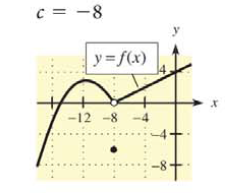Chapter 9.1, Problem 5E### Mathematical Applications for the ...

11th Edition
Ronald J. Harshbarger + 1 other
ISBN: 9781305108042

#### Solutions

Chapter
Section### Mathematical Applications for the ...

11th Edition
Ronald J. Harshbarger + 1 other
ISBN: 9781305108042
Textbook Problem

# In Problems 1-6, a graph of y = f(x) is shown and a c-value is given. For each problem, use the graph to find the following, whenever they exist.(a) lim x → c f ( x ) and (b) f ( c ) c = − 8(a)

To determine

The value of limxcf(x) by the use of the graph,Explanation

Given Information:

The value of c is 8. The graph of y=f(x) is,

Explanation:

Consider the provided graph,

The value of c is equal to 8.

The limit from the left is represented by limx8f(x) and the limit from the right is represented by limx8+f(x). The limxcf(x) will exist at c=8 when the limit from the left, that is, the values of f(c)<

(b)

To determine

The value of f(c) by the use of the graph,### Still sussing out bartleby?

Check out a sample textbook solution.

See a sample solution

#### The Solution to Your Study Problems

Bartleby provides explanations to thousands of textbook problems written by our experts, many with advanced degrees!

Get Started

#### Find more solutions based on key concepts